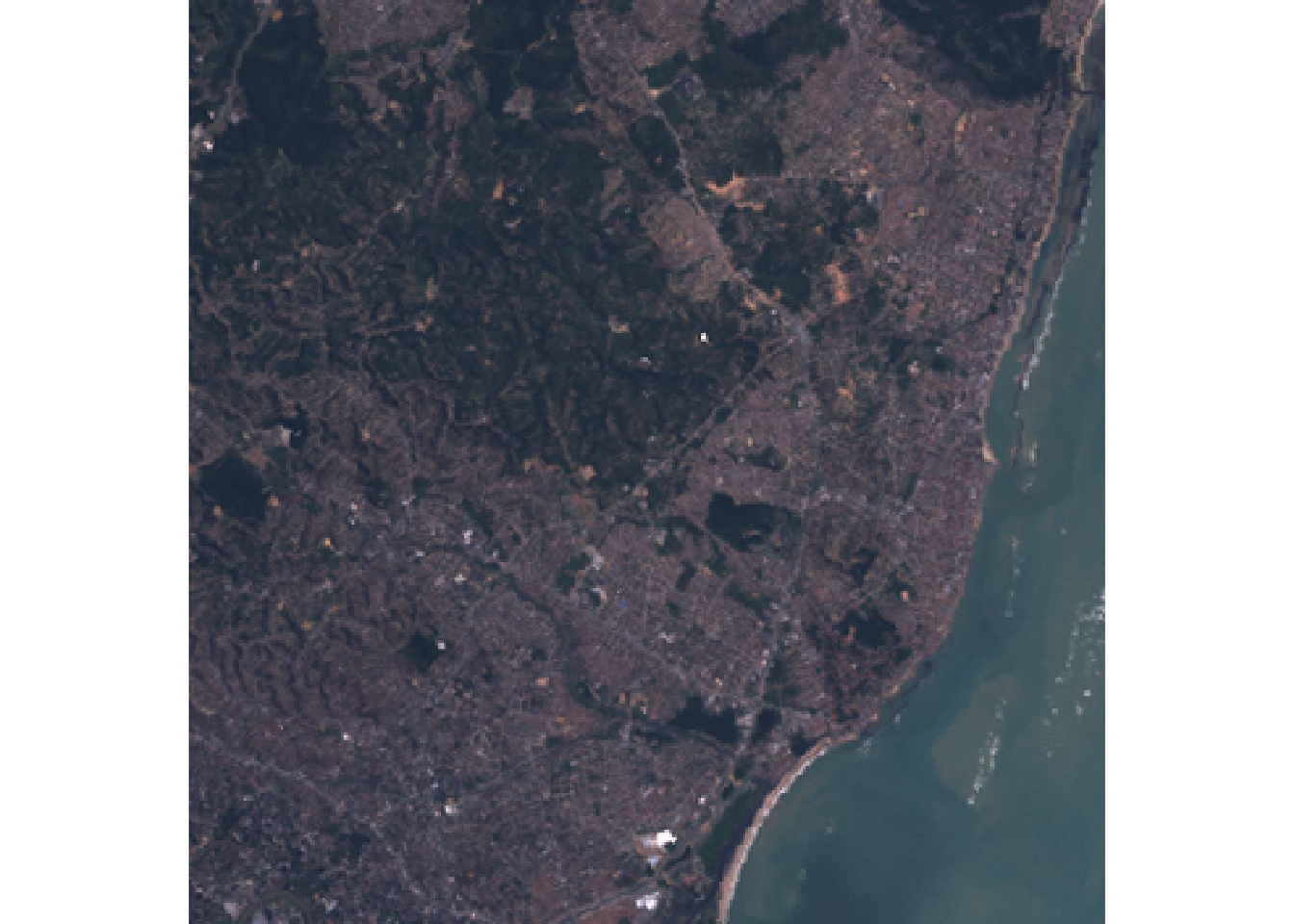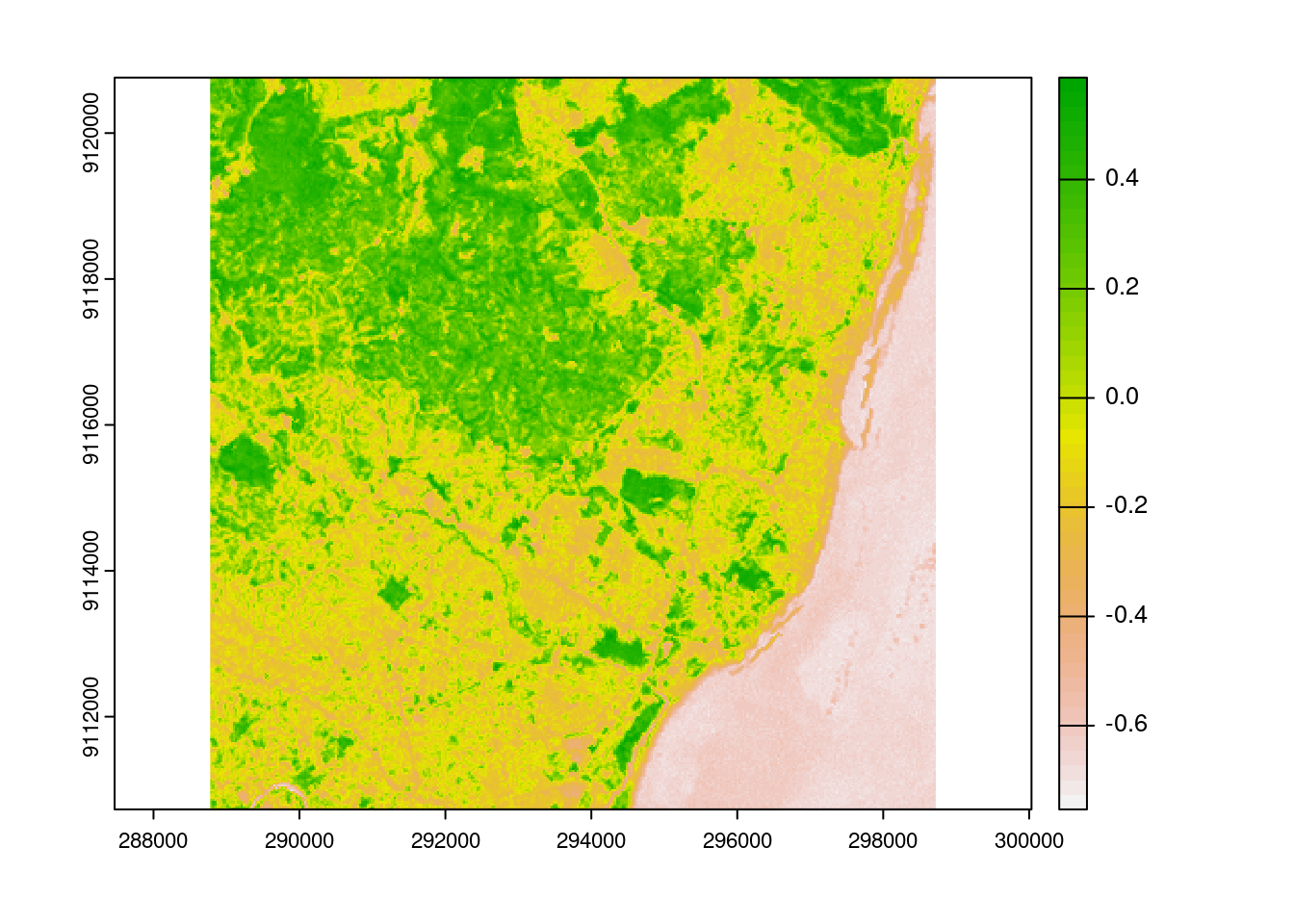Last Update: 7 October 2022

## Overview

A common approach to distribute the workload in raster calculations is to split a large raster into smaller ‘tiles’ or ‘chunks’. This tutorial shows how to speed up a NDVI calculation by creating tiles and then distributing the tiles across cores. This process is not specific to calculating NDVI. You can use this approach with any calculation that is performed per cell/pixel without depending on the data in neighboring pixels (if neighboring data is needed, extra steps are involved).

This tutorial assumes you are running this Rmarkdown file in RStudio Server. The easiest way to do that is with Open OnDemand (OoD) on Ceres or Atlas. Select the following parameter values when requesting a RStudio Server app to be launched depending on which cluster you choose. All other values can be left to their defaults. Note: on Atlas, we are using the development partition so that we have internet access to download files since the regular compute nodes on the atlas partition do not have internet access.

Ceres:

• Slurm Partition: short
• R Version: 4.2.0
• Number of cores: 16
• Memory required: 24G

Atlas:

• R Version: 4.1.0
• Partition Name: development
• QOS: normal
• Number of tasks: 16
• Additional Slurm Parameters: –mem=24G

To download the Rmarkdown file for this tutorial to either cluster within OoD, you can use the following lines:

library(httr)
tutorial_name <- 'GRWG22_RasterTiles.Rmd'
GET(paste0('https://geospatial.101workbook.org/tutorials/',tutorial_name),
write_disk(tutorial_name))


In this tutorial, parallelization is performed within R using the resources allocated to the launched RStudio Server session. If you are interested in seeing an example on how to submit your own SLURM job, please see this tutorial.

Language: R

Primary Libraries/Packages:

terra Spatial Data Analysis https://cran.r-project.org/web/packages/terra/index.html
foreach Provides Foreach Looping Construct https://cran.r-project.org/web/packages/foreach/index.html
doParallel Foreach Parallel Adaptor for the ‘parallel’ Package https://cran.r-project.org/web/packages/doParallel/index.html

## Nomenclature

• Parallel processing: Distributing computational tasks among multiple cores.
• Core: A processor on a central processing unit; a physical or logical component that can execute computational tasks.
• Tile (or chunk): A continuous segment of a raster dataset or multi-dimensional array.
• NDVI: Normalized Difference Vegetation Index, a quantity derived from the red and near-infrared bands of imagery to detect vegetation.

## Analysis Steps

• Open Imagery and Setup Tiles - Open a GeoTIFF of Landsat 7 imagery and divide it into multiple smaller images.
• Define NDVI function - Write a function to calculate Normalized Difference Vegetation Index from an image file.
• Compare serial versus parallel computation times - Measure the time it takes to perform the NDVI calculation across tiles in serial and in parallel
• Option 1: using the foreach and doParallel packages
• Option 2: using the parallel package
• Visualize NDVI results - View the NDVI values in tiles as a whole image.

## Step 0: Import Libraries/Packages

For this tutorial, we will use the stars package for one of its example datasets, the terra package for handling raster data, and the doParallel and foreach packages together for distributing our calculations. The stars and terra packages are each available in the R site library for RStudio Server on Ceres and Atlas Open OnDemand, though not in the R site library if you were to load an R module in a shell. However, the terra version on Atlas is not a recent enough version for this tutorial, so we will install the latest version. As of this writing, foreach and doParallel are not in the site library and will need to be installed if you have not already done so. To learn more about installing packages on Ceres, see this guide.

install.packages(c('foreach','doParallel','terra'))

library(terra)
library(foreach)
library(doParallel)


## Step 1: Open Imagery and Setup Tiles

### Step 1a: Open imagery

The imagery file we will use is available from the stars package. It is an example image from Landsat 7 and you can learn more about it by calling ?L7_ETMs. In that documentation, it indicates that the first three bands are from the visible part of the electromagnetic spectrum (blue, green, red) and the fourth band in the near-infrared band. We can use terra’s function rast to read the imagery file. The plotRGB function will let us visualize the image in typical RGB. We can see that it is of a coast with forested areas that should have relatively high NDVI values.

L7_ETMs <- rast(system.file("tif/L7_ETMs.tif", package = "stars"))

# RGB Image using the third-first bands
plotRGB(L7_ETMs[[3:1]])This image is fairly small and we do not actually need to speed-up the NDVI calculation with parallel processing. So we will make it artificially a big data problem by disaggregating the pixels, i.e. making a greater number of smaller cells, for the sake of a portable example. The function disagg accepts a raster object and a disaggregation factor, i.e. the number of cells to create from each original cell in each direction. Below, we are using a factor of 20 which will create 400x pixels than the original.

disagg_factor <- 20
nir_red_fine <- disagg(L7_ETMs[[3:4]], disagg_factor,
filename = "nr_test.tif",
overwrite = TRUE)


### Step 1b: Making tiles

The terra package comes with a handy function for making raster tiles called makeTiles to which you pass the raster you want to split up, a template raster indicating how to split it up into tiles, and how to name the resulting files containing the tiles. First, we will specify how many tiles we want. This example will use 4 tiles in each directions for a total of 16 tiles. Next, we will create an empty raster template will the number of rows and columns matching our number of tiles and our image’s spatial extent and CRS. The we call makeTiles which will write the tiles to file and return the filenames.

# Set number of tiles (number of rows, number of columns)
num_tiles <- c(4,4)

# Define empty raster template to define tiles
tile_template <- rast(nrows = num_tiles,
ncols = num_tiles,
crs = crs(nir_red_fine),
ext = ext(nir_red_fine))

# Generate the tile files using the red and NIR bands only
nr_tiles <- makeTiles(nir_red_fine,
tile_template,
filename="tile_.tif",
overwrite=TRUE)


## Step 2: Defining NDVI function

Next, we want to define a function that will calculate NDVI for the pixels in one tile. The function will accept a tile’s filename, open that file with terra, calculate NDVI for the pixels, then write the resulting single-band raster to a new file.

# Define NDVI function that writes results to file
normalized_diff_r <- function(fname){
nr <- rast(fname)
NDVI_r <- (nr$L7_ETMs_4 - nr$L7_ETMs_3)/(nr$L7_ETMs_4 + nr$L7_ETMs_3)

writeRaster(NDVI_r, filename=paste0('NDVI_',fname), overwrite = TRUE)
}


## Step 3: Comparing serial and parallel processing times

To have a baseline to which to compare how long the parallel approach takes, we can first time the serial approach. The base R system.time() function provides basic timing capabilities and reports three different timings: user, system, and elapsed. The third timing, elapsed, is the physical or ‘wall’ time elapsed and the one we are interested in comparing among our approaches.

### Step 3a: foreach/doParallel

#### Serial

In this first option, we are using the foreach package as a serial backend that the doParallel extends with parallel processing. Using foreach is similar to using a for loop with a slightly different syntax in the first line:

• for is replaced with foreach
• in is replaced with =
• ){ is replaced with ) %do% {
# for loop
for(i in 1:N){
}

# foreach
foreach(i = 1:N) %do% {
}


To have NDVI calculated for each of our tiles, we will have foreach iterate over each tile filename (which was returned by makeTiles) and call our normalized_diff_r function for each iteration.

# Time the serial execution
system.time({
foreach(fname=nr_tiles) %do% {
normalized_diff_r(fname)
}
})

   user  system elapsed
10.277   0.296  12.665


#### Parallel

To use doParallel to parallelize foreach, two things must be done:

1. You register doParallel with the number of cores you want to use
2. You change %do% to %dopar% in your foreach statement

Here, we are using as many cores as number of tiles so each core will have one task to complete.

# Register doParallel
registerDoParallel(cores = prod(num_tiles))

# Time the parallel execution
system.time({
foreach(fname=nr_tiles) %dopar% {
normalized_diff_r(fname)
}
})

   user  system elapsed
9.144   1.426   8.189


### Step 3b: parallel

There is another similar approach using the parallel package, which comes with base R. Instead of extending foreach, we will see how parallel extends base R’s lapply with mclapply.

#### Serial

Again, we will first time how long the serial lapply approach takes to perform our NDVI calculation. lapply accepts a vector of inputs (our tile filenames) and a function that accepts those inputs (normalized_diff_r).

# Time the serial execution
system.time({
lapply(nr_tiles, normalized_diff_r)
})


   user  system elapsed
10.302   0.260  12.746


#### Parallel

To use the parallel version of lapply, two changes are made to our code above:

1. Call mclapply instead of lapply
2. Pass the desired number of cores

Like with doParallel, we are requesting as many cores as the number of tiles.

# Time the parallel execution
system.time({
mclapply(nr_tiles, normalized_diff_r, mc.cores = prod(num_tiles))
})


   user  system elapsed
6.226   1.213   7.670


## Step 4: Visualize NDVI results

There is a terra function vrt (Virtual Raster Tiles) for visualizing tiles without having to mosaic first. Note: if you are familiar with using GDAL (Geospatial Data Abstraction Library), their VRT is a more general virtual format not specific to just tiling.

We can see from the image that the forested areas have the higher NDVI values as we expected.

virtual_ndvi <- vrt(paste0('NDVI_',nr_tiles))
plot(virtual_ndvi)### Continue to explore

Things you can change in this tutorial to see how it affects the difference in processing time between serial and parallel (or the two parallel library options):

• The disaggregation factor
• The number of tiles
• The number of cores